HOME plateWON | World!OfNumbers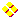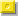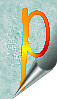Palindromic Primes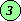Page 3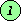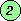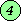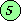Circular PrimesHome PrimesUndulating PrimesSums of PrimesPalindromic Primes

Palindromic Prime Pyramid Puzzle
by G. L. Honaker, Jr. [ January 20, 2000 ]

2
727
37273
333727333
93337273339
309333727333903
1830933372733390381
92183093337273339038129
3921830933372733390381293
1333921830933372733390381293331
18133392183093337273339038129333181
***
***
***

The puzzle is quite simple in fact.
Can you extend the pattern beyond these 11 rows upto let's say 727 (cf. row 2) or even 929 ?
Note that each term is the smallest to have the previous term as a centered substring,
beginning with the smallest palindromic prime 2.

Garland and I believe that working on this problem is the best cure for 'aibohphobia'
or 'fear of palindromes'...

Here is Carlos Rivera's comment from [ January 22, 2000 ]

I have started getting the solution to this sequence. Inside the capabilities
of UBASIC this sequence can be obtained only up to 400 rows or so... why ?
I'm now at row 100 and the number is 563 digits large (of course that I'm only
printing the additional substring from one number to the other, and also the
quantity of digits of each number).
Then the target of 929 rows is out of possibilities to any known public code
available: this number (929 rows) must be no less than 4500 digits large,
if it exists...

Needless to say that all the numbers in my sequence are, by the moment
"strongpseudoprime". The bad news is that any mistake in any step spoils the
rest of the numbers... so, this is a very hard puzzle in time consumption
terms, before we can be sure to say that all the members are palprimes.
Watch it!...

And here is Carlos Rivera's message from the day after [ January 23, 2000 ]

Good sunday. I have stopped my search at 1001 digits.
Now the search has begun to be slow. I let other (brave) people continue...

```row	extreme	total
number	left	digits

1       2       1
2       7       3
3       3       5
4       33      9
5       9       11
6       30      15
...
158     1156    957
159     1011    965
160     38      969
161     1156    977
162     1070    985
163     1419    993
164     1459    1001
```
If you're interested, the complete sequence can be consulted via the "VIEW | SOURCE"
menu-function of most modern browsers applied to this very page (see then list at the end of that page).

[ August 27, 2000 ]
Chris Caldwell and G. L. Honaker, Jr. wrote an article that soon will be publicized
into the "Journal of Recreational Mathematics". You don't have to wait for the postman
to come by as a preview of their discussion about these types of pyramids can be found
Supplement to "Palindromic Prime Pyramids"
Palindromic prime pyramids - reference
Prime Curios! 18133...33181 (35 digits)

References : Sloane's Integer Sequences
A034276 - Smallest prime that generates a prime pyramid of height n.
A046210 - Smallest palindromic prime that generates a palindromic prime pyramid of height n.
A047076 - a(n+1) is the smallest palindromic prime containing exactly 2 more digits on each end than the previous term, with a(n) as a central substring.
A052091 - Left parts needed for the construction of the palindromic prime pyramid starting with 2.
A052092 - Lengths of the palindromic primes from Honaker's sequence A053600.
A052205 - a(n+1) is smallest palindromic prime containing exactly 3 more digits on each end than the previous term, with a(n) as a central substring.
A053600 - a(n+1) is the smallest palindromic prime with a(n) as a central substring.
A082563 - Prime pyramid starting with 3. (by Benoit Cloitre [ May 5,2003 ] ).

3
131
11311
121131121
1212113112121
36121211311212163
303612121131121216303
7230361212113112121630327
30723036121211311212163032703
723072303612121131121216303270327
1472307230361212113112121630327032741
114723072303612121131121216303270327411
7711472307230361212113112121630327032741177
1237711472307230361212113112121630327032741177321
***
***
***

```

```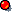Patrick De Geest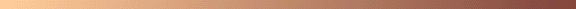13331  is the smallest palprime with the following property :
Inserting any digit d between adjacent digits of this palprime never produces a new prime !
There are ten different single digits so let us check them out each in turn.
103030301 = 11 x 29 x 71 x 4549
113131311 = 3 x 17 x 37 x 167 x 359
123232321 = 23 x 2239 x 2393
133333331 = 11287 x 11813
143434341 = 3 x 3 x 3 x 293 x 18131
153535351 = 1997 x 76883
163636361 = 7 x 23376623
173737371 = 3 x 4517 x 12821
183838381 = 13469 x 13649
193939391 = 79 x 2454929
It shouldn't be too hard to find a few more like palprimes ! Please submit them to me.
In case this assignment is too easy for you...
what about finding the first palprime with this counter-property :
Inserting any digit d between adjacent digits of palprime P always produces a new prime !
Take this opportunity to become eternally praised and find this elusive palprime P !
Your name will always be linked with this extraordinary number, if it exists of course !
My best shot up to now [ October 3, 1999 ] is this '6 out of 10' solution with palprime 131,
but was quickly superseeded by Carlos Rivera's '7 out of 10' 13-digit solution.
10301 = prime
11311 = prime
12321 = 3 x 3 x 37 x 37
13331 = prime
14341 = prime
15351 = 3 x 7 x 17 x 43
16361 = prime
17371 = 29 x 599
18381 = 3 x 11 x 557
19391 = prime
Thanks to Carlos Rivera we now have this '7 out of 10' solution !
7762868682677_0 = prime
7762868682677_1 = 29 x 401 x 10038749783 x 61432020031
7762868682677_2 = prime
7762868682677_3 = 11 x 9411043 x 123490751 x 576788519
7762868682677_4 = prime
7762868682677_5 = prime
7762868682677_6 = prime
7762868682677_7 = prime
7762868682677_8 = prime
7762868682677_9 = 10987 x 33599 x 21616267514286769
On [ October 21, 1999 ] Carlos Rivera communicated a second '7 out of 10'
of 19 digits : 1009074444444709001
This still means we need an '8 out of 10', a '9 out of 10' and a '10 out of 10' solution !
0 out of 10 = 13331
1 out of 10 = 101
2 out of 10 = 383
3 out of 10 = 151
4 out of 10 = 11311
5 out of 10 = 11 (was first 353 but Jim Howell corrected this)
6 out of 10 = 131
7 out of 10 = 7762868682677 by Carlos Rivera [ October 20, 1999 ]
8 out of 10 = ?
9 out of 10 = ?
10 out of 10 = ?
Enjoy the search !
Solutions may also be forwarded to my friend Carlos Rivera (email)
Please visit his PP&P puzzle 72 on Persistent Palprimes.
Featured in Prime Curios! 13331

This paragraph deals with another yet very unique palindromic prime.
The number in question is 134757431
It can be expressed as a ninedigits sequence raised to the power of another combination of the ninedigits sequence.
And that in exactly three different ways. No other palindrome (even a composite one) exists with that property !

134757431 17 + 23 + 38 + 45 + 54 + 62 + 71 + 89 + 96 17 + 25 + 38 + 41 + 52 + 64 + 73 + 89 + 96 17 + 28 + 34 + 42 + 53 + 65 + 71 + 89 + 96

Another unique palindrome prime exists that is expressible like explained above but only in a twofold manner.
You like math puzzles ? Ok, then find that palindromic prime !

Some smallest primes that turn out to be palindromic !

The smallest prime of length 7 containing only the digits 7 and 8 is palindromic
7778777

The smallest prime of length 9 containing only the digits 3 and 5 is palindromic
333535333

My highest esteem for the person who can find an example with digitlengths beyond 9 !
Source : "Sloane's Integer Sequences" A036929 up to A036951 (P. De Geest).

The smallest prime containing exactly eleven 6's is palindromic (P. De Geest)
1666666666661
[See Sloane's A037065.]
Featured in Prime Curios! 1666666666661
```
```Carlos RiveraJaime AyalaMagic Squares composed of only palprimes

A collaboration of Carlos Rivera and Jaime Ayala turned out to be very fruitful as after
two or three weeks of exhaustive searching they came up with the following two extraordinary
Magic Squares [ May 22 and May 24,1999 ]. Quoting

"As far as our search is correct these two are the smallest Magic Squares with palprimes.
We found no solutions with 3, 5, 7 or 9 digits and no other with 11 digits.
"

Due to the work of Jean Claude Rosa [ March 31, 2002 ]
we know that Rivera's and Ayala's Magic Squares are not the smallest ones.
For all the details see WONplate 129.

109155519011213353312111527872511Magic
Square I
121379731211152565251110913331901all numbers are
palprimes
115234325111091777190112135753121by
Rivera & Ayala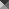107977797011433606334112568586521Magic
Square II
143382833411256747652110796669701all numbers are
palprimes
125663665211079888970114337173341by
Rivera & Ayala

Carlos Rivera from Nuevo León, México constructed the following nice series [ May 26, 1998 ],
displaying sums of three consecutive palindromic primes that are also palindromic primes.
Many more no doubt can be found. Select the most beautiful ones and send them to me, please !

```101 + 131 + 151 = 383
30103 + 30203 + 30403 = 90709
31013 + 31513 + 32323 = 94849
1221221 + 1235321 + 1242421 = 3698963
102838201 + 103000301 + 103060301 = 308898803
110111011 + 110232011 + 110252011 = 330595033
111010111 + 111020111 + 111050111 = 333080333
133020331 + 133060331 + 133111331 = 399191993
302313203 + 302333203 + 302343203 = 906989609
323222323 + 323232323 + 323333323 = 969787969 (*)
11021312011 + 11021412011 + 11022122011 = 33064846033
12301010321 + 12303230321 + 12303730321 = 36907970963
13002220031 + 13002420031 + 13004040031 = 39008680093
13100100131 + 13100300131 + 13101410131 = 39301810393
```
Note that the middle number of the sum (*) is a special number !
It is in fact an undulating palindromic prime.

The next trio of consecutive palindromic primes - 43 digits - is impressive...

 1000000000000002109952599012000000000000001 + 1000000000000002110000000112000000000000001 + 1000000000000002110025200112000000000000001 = 3000000000000006329977799236000000000000003
The above and following examples are the smallest possible ones for that given length because they start form a "zero nut" (the "nut" is the central number before "reflecting" it to get the central palindrome condition and at the same time the overall palindrome condition... do you follow me?) and the nut is then increased one by one until the three palprimes added yield another palprime.

... but still nothing compared to these awesome - 99 and 191 digits - constructions (both by Carlos Rivera) !
Note, it took Carlos one week to get the 191 (itself also a palprime!) solution with his code !

 1(0)423332222222333(0)421 + 1(0)423332253522333(0)421 + 1(0)423332304032333(0)421 = 3(0)429996779776999(0)423

 1(0)87132298010892231(0)871 + 1(0)87132300858003231(0)871 + 1(0)87132301111103231(0)871 = 3(0)87396899979998693(0)873

[ June 10, 2003 ]
Jens Kruse Andersen (email) found a much larger solution (515 digits).
Its palindromic length is just a bonus!

For the strategy of his clever approach I refer to Carlos Rivera's webpage at http://www.primepuzzles.net/puzzles/puzz_023.htm.

 1(0)243101031223000000000322130101(0)2431 + 1(0)243101031223000313000322130101(0)2431 + 1(0)243101031223008545800322130101(0)2431 = 3(0)243303093669008858800966390303(0)2433

Splendid solution, Jens. Well done!

Exist there a solution with carries occurrence ?

Carlos Rivera discovered that palindromic prime 71317
is expressible as the sum of consecutive primes in three ways [ August 9, 1998 ].

71317 2351 + 2357 + ..... + 2579 + 2591 ( 29 primes ) 10163 + 10169 + 10177 + 10181 + 10193 + 10211 + 10223 ( 7 primes ) 14243 + 14249 + 14251 + 14281 + 14293 ( 5 primes )

Notice that the primes involved in each equation are different,
and that the quantity of primes in each equation is also a prime number.
Featured in Prime Curios! 71317

```
```Enoch HagaPalprimes in the 37th Mersenne prime

Enoch Haga, author of the book "Exploring Primes on Your PC", 2nd edition 1998,
is fascinated by the largest known prime (23021377–1) i.e. the 37th Mersenne prime with 909526 digits.
M37 =  12741168303009336743355421517673... ...685875891125668064038159285739505469
He discovered [ February 11, 1999 ] that the Number of the Beast 666 occurred 787 times (and 787 is a palprime).
Another nice palprime 313 - occurring 903 times !! - shows up also on the right side of the sum
909526 + 787 = 910313.
[ G. L. Honaker, Jr. remarks that 37 x (6 + 6 + 6) = 666 ! ]
See the section 'Enoch Haga' for the story behind the mysterious number 313 at page.
Facts and Figures
The smallest palprime NOT occurring in M37, beginning with 2, is 1003001.
1082801 is the first 7-digit palprime to be found in M37 exactly ONE TIME.
```
```G. L. Honaker, Jr.Beasty palprimes in the 37th Mersenne prime

G. L. Honaker, Jr. following the work of Enoch Haga at close quarters recalls that
16661 occurs exactly 11 times in the 37th Mersenne prime
16661 is the 1928th prime
and not only that, but also that the digitaddition of these two numbers are equal
1 + 6 + 6 + 6 + 1 = 1 + 9 + 2 + 8
Featured in Prime Curios! 16661

P. De Geest draws the attention to the following 'base 12' fact :
16661{12} is the square of 12B{12} or in decimal 32041 = 1792 !

The largest probable primes with 16661 as the negative displacement
from its power of ten axis are the following two so far
10871416661
101237916661

Trinity of the Beast

One day I wrote an email to a group of people and presented them the sum of the first 666 palindromic primes
namely 2391951273 and asked them if they could relate this to the Number of the Beast.
Very soon afterwards G. L. Honaker, Jr. came up with what he calls the 'Trinity of the Beast' :

The sum of the first 666 palindromic primes = 2391951273 [De Geest]23 + 33 + 93 + 13 + 93 + 53 + 13 + 23 + 73 + 33
= 666 + 666 + 666 [Honaker]

Note that 3 x 666 = 1998 is the year in which G. L. Honaker, Jr. made this inspired discovery !

And he continues :

9230329 is the 666 th palindromic prime number.
Featured in Prime Curios! 9230329
9+2+3+0+3+2+9 = 28 (the second perfect number... following 6)
28 = 13 + 33 ... more Trinity of the Beast ?
Note the 3 middle digits, 303, is the number of primes less than or equal to 1999
1999 being the first prime of the form 666n+1 !

Honaker's Constant = 1.3239820264...

G. L. Honaker, Jr. kindly lends his name to the following 'convergent' infinite series
that is the sum of the reciprocals of all the palindromic primes.
Featured in Prime Curios! 1.32398...

1
2
+ 1
3
+ 1
5
+ 1
7
+ 1
11
+ 1
101
+ 1
131
+ ... = ?

What is the value of this series ? Garland's very first guess was the square root of two.
This soon turned out not to be the case. Mike Keith came to the rescue as he computed
the value of the sum up through all 11-digit palindromic primes. The value he got was :

 1.3239820264...

Mike commented further

"I am sure the first 5 digits after the decimal point have been determined (so for sure it's 1.32398...)
and I think it's very likely the next digit is correct (so we know it's 1.323982...) but after that I can't
be sure. I would need to add the 13-digit palprimes and see how that result differs in order to be
absolutely sure how many of these digits are correct."

Finally Garland found a nice approximation using a fraction with palprime numerator and denominator :

96269 / 72727 = 1.3237037... correct to three decimal places.

[See more at Sloane's A046852 and A046853.]

Some beautiful palprimes

( This section is best read from bottom to top ! )

923032900000000000000000000000000000000666000000000000000000000000000000009230329
A palprime of length 81 ! [ April 14, 1999 ]
Note 9230329 is the 666th palindromic prime.
666 is flanked by 32 zero's on each side
and 666 x 32 = 21312 a nice palindrome...
or 666 x 64 = 42624 another nice palindrome...
Featured in Prime Curios! 9230329

31415926535897932384626433833462648323979853562951413
Another beautiful palprime by G. L. Honaker, Jr. [ March 13, 1999 ]
Using his own terminology we call this a Pi-lindromic prime !
This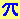-lindromic prime has 53 digits. [Sloane's reference A039954 and A119351.]
Featured in Prime Curios! 31415...51413 (53-digits)

151515151515151
A smoothly undulating palindromic prime (SUPP) containing 15 digits. [ March 10, 1999 ]
Hmmm... the smallest 'mnemonic' prime ?
Featured in Prime Curios! 151515151515151

10080010080010080010080010080010080_
01008001008001008001008001008001008_
00100800100800100800100800100800100_
80010080010080010080010080010080010_
08001008001008001008001008001008001

A 175-digit tetradic palprime discovered by G. L. Honaker, Jr. on [ March 9, 1999 ]
Featured in Prime Curios! 10080...08001 (175-digits)

313_0{150}_1183811_0{150}_313
The smallest(?) palprime of length 313 starting and ending with 313. [ March 7, 1999 ]
Note that both 313 and 1183811 are palindromic reflectable primes - a Sloane A007616 class !
Clearly G. L. Honaker, Jr. is fascinated with triadic (or 3-way) primes i.e. which are invariant upon
reflection only along the line they are written on, so the digits may be 0, 1, 3 and 8.
Featured in Prime Curios! 31300...00313 (313-digits)

7_0{48}_666_0{48}_7
A palprime with a palprime total of digits namely 101 this time ! [ March 6, 1999 ]

31300000000000000000000000006660000000000000000000000000313
A nice palprime of length 59 sent by G. L. Honaker, Jr. on [ February 28, 1999 ]
See the section 'Enoch Haga' for the story behind the mysterious number 313 at page.
And yes we all know that 666 is the famous number of the beast...

```

```Mike KeithThe following find of G. L. Honaker, Jr. is a by-product of his sequence A036978 dd. [ January 17, 1999 ].
Start with a prime (that happens to be palindromic in this case) e.g. 373.
Featured in Prime Curios! 373
Each next term describes the previous terms according to the rules stipulated in A005150.
The puzzle statement is now to find the largest series of primes.
Our example is the only 3-digit number (a palprime at that!) producing 3 more primes in a row !
373 palprime
131713 prime
111311171113 prime
311331173113 final prime of length 12.
1321232217132113 composite!!
"There may be better 'generators' out there... this is as far as I have taken it." G. L. Honaker, Jr. wrote.

Carlos Rivera saw this puzzle on 'descriptive primes' and went also to work - See Puzzle 36.
He reported to me [ January 22, 1999 ] that he found a series of six (6) terms that are primes.
[ See Sloane A037033 - A038131 - A038132 ]
Featured in Prime Curios! 233
233 prime
1223 prime
112213 prime
21221113 prime
1211223113 prime
11122122132113 final prime of length 14.
312211221113122113 composite!!

This one sent in by Carlos the day after [ January 23, 1999 ]
120777781 prime
111210471811 prime
311211101417111821 prime
13211231101114111731181211 prime
111312211213211031143117132118111221 prime
31131122211211131221101321141321171113122118312211 final prime is 50 digits long!

Later on [ January 24, 1999 ] Carlos found yet another generator yielding a prime of 52 digits still as a sixth term.
402266411 prime
141022261421 prime
11141110321611141211 prime
31143110131211163114111221 prime
132114132110111311123116132114312211 prime
1113122114111312211031133112132116111312211413112221 final prime

Mike Keith has found three more length-6 prime chains [ February, 1999 ]
Here are the beauties !

starting at prime 1171465511 and ending with a 54-digit prime :
21171114162521 prime 2
1221173114111612151211 prime 3
11222117132114311611121115111221 prime 4
21322117111312211413211631123115312211 prime 5
121113222117311311222114111312211613211213211513112221 final prime 6

starting at prime 1623379207 and ending with a 74-digit prime :
111612231719121017 prime 2
311611221311171119111211101117 prime 3
1321162122111331173119311231103117 prime 4
111312211612112231232117132119132112132110132117 prime 5
31131122211611122122131112131221171113122119111312211211131221101113122117 final prime 6

starting at prime 1955771683 but ending with a smaller 68-digit prime :
1119252711161813 prime 2
311912151217311611181113 prime 3
132119111211151112111713211631183113 prime 4
111312211931123115311231171113122116132118132113 prime 5
31131122211913211213211513211213211731131122211611131221181113122113 final prime 6

Mike Keith predicts a length-7 chain should occur around 10^11, after having made some calculations
about the relative probability to find chains of this type of different length.

[ November 2002 ]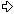This is not the end of the story ! An exhaustive search was done by Walter Schneider.
Currently the search has arrived at starting number 10^11. In total 59 sequences of
length 6 are found and one sequence of length 7 starting at 19972667609.
So far no sequence of length 8 or more is known.

```

```

Contributions

Carlos Rivera (email)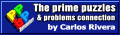from Nuevo León, México and Jaime Ayala (email) - go to topic

Enoch Haga (email) for his discoveries of palindromes in the 37th Mersenne prime - go to topic

G. L. Honaker, Jr. (email) from Bristol, Virginia made a beastly interesting discovery - go to topic

Mike Keith (email) found many of the larger self-descriptive primes- go to topic

```

```

[TOP OF PAGE]

Patrick De Geest - Belgium- Short Bio - Some Pictures